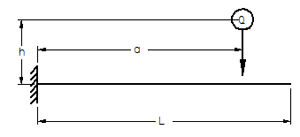Related Resources: calculators

### Stress from Shock Load Fixed End Equations and Calculator Impact Load

Impact Stress from Drop Load onto Beam Fixed on one End and Struck at the Other End Equations and Calculator. Stresses produced by shock impact loads.or, approximatelyWhere:

Q = Weight of dropped mass (N)
E = Modulus of Elasticity (N/mm2)
I = Area Moment of Inertia (mm4)
L = Length of beam (mm)
a = Distance from support (mm)
h = Height distance (mm)
p = Stress (N/mm2)

Stresses in Beams Produced by Shocks.

Reference:

Machinery's Handbook, 27th Edition Stresses Produced in Beam by Shocks